Proportional Relationships

Proportions

True Proportions
• A proportion is an expression of the equality of two ratios or rates.
• A proportion is true if the fractions are equal when written in lowest terms.

Example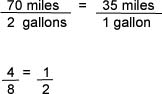• Any units expressed in a proportion must be consistent. (When units are used, the units of the numerators are the same, and the units of the denominators are the same.)
• In any true proportion, the "cross products" are equal.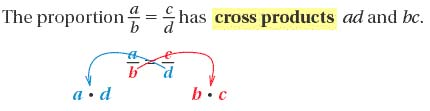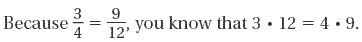Solving Proportions

Sometimes one of the numbers in a proportion is unknown. In this case, it is necessary to solve the proportion.

To solve the proportion, find a number to replace the unknown so that the proportion is true. You can use cross-products to solve a proportion.

Example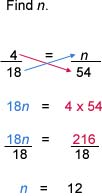Proportions are helpful when solving problems that require for a scale or rate to be followed.

When writing a proportion to solve a problem, be sure to use follow a consistency of units.

Example:

A bakery makes two kinds of pies: cherry and apple. The ratio of cherry pies to apple pies is 5 to 9. If there are 70 pies in all, how many are cherry?

Strategy:

Step 1: Determine the ratio of cherry pies to total pies.

Step 2:  Set up a proportion of cherry pies to total pies to find the number of cherry pies at the bakery.

Solution:

Step 1: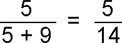For every 14 pies, 5 are cherry.

Step 2: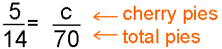5 x 70 = 14 c      Cross products property.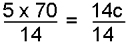Divide each side by 14.

25 = c            Simplify.

There are 25 cherry pies at the bakery.

Practice

1.)   Use cross products to determine whether each proportion given below is a true proportion.

a.)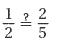b.)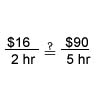c.)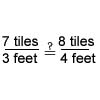d.)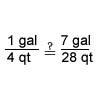2.) Solve each proportion. Round to the nearest hundredth if needed.

a.)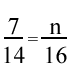b.)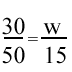c.)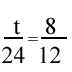d.)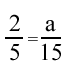e.)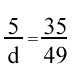3.)  Solve the following problems using a proportion.

a.)  The dosage of a certain medication is 2 ounces for every 50 pounds of body weight.  How many ounces of medication are required for a person who weighs 175 pounds?

b.)  Three tablespoons of fertilizer are added to every 4 gallons of water.  How many tablespoons of fertilizer are required for 10 gallons of water?

c.)  The scale on a map is 1.25 inches equals 10 miles.  How far apart in miles are two towns that are 2 inches apart on the map?

d.)  A contractor charges \$9.25 per square foot for stucco work.  A homeowner was given the estimate of \$5,550 for stucco work on her home.  What was the square footage used for the estimate?

1.)

a.) not true

b.) not true

c.) not true

d.) true

2.)

a.) n = 8

b.) w = 9

c.) t = 16

d.) a = 6

e.) d = 7

3.)

a.) 7

b.) 7.5

c.) 16 miles

d.) 600